ProofCustom SearchPROOF: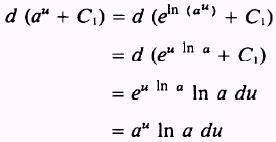so that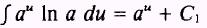But In a is a constant, so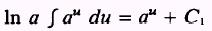Then, by dividing both sides by In a: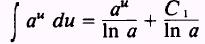orwhere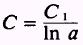EXAMPLE: EvaluateSOLUTION: Let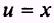so that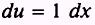Therefore, by knowing thatand using substitution, we find thatEXAMPLE: Evaluate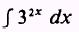SOLUTION. Letso thatThe integral should contain a factor of 2. Thus we insert a factor of 2 in the integral and compensate by multiplying the integral by 1/2. Then,Therefore,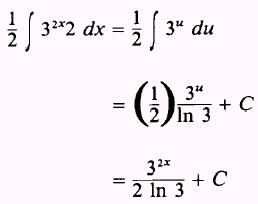EXAMPLE: EvaluateSOLUTION: Let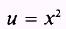so thatWe find we need 2x dx; therefore, we remove the 7 and insert a 2 by writing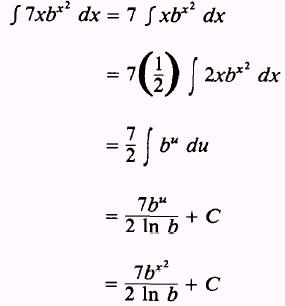PRACTICE PROBLEMS: Evaluate the following integrals: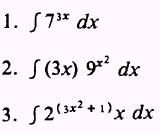ANSWERS:Integrated Publishing, Inc. - A (SDVOSB) Service Disabled Veteran Owned Small Business